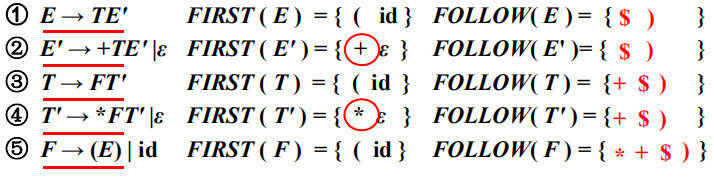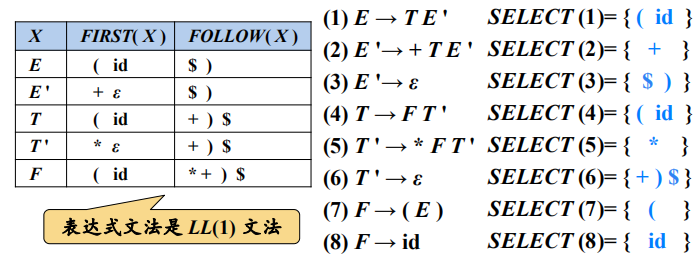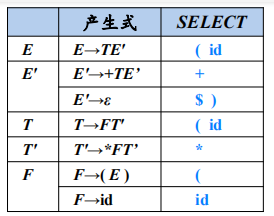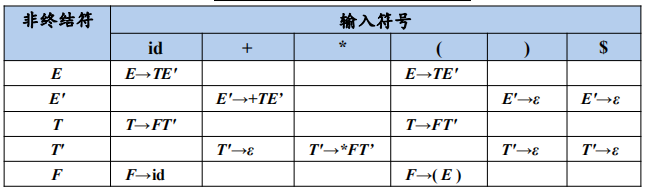### 计算文法符号X的FIRST(X )

• FIRST ( X )：可以从X推导出的所有串首终结符构成的集合
• 如果 X => * ε，那么 ε∈FIRST ( X )

① E → TE' FIRST ( E ) = { ( id }
② E' → +TE' |ε FIRST ( E' ) = { + ε }
③ T → FT' FIRST ( T ) = { ( id }
④ T' → FT' |ε FIRST ( T' ) = { ε }
⑤ F → (E)|id FIRST ( F ) = { ( id }

### 算法

• 如果X是一个终结符，那么FIRST ( X ) = { X }
• 如果X是一个非终结符，且 X→Y1…Yk∈P (k≥1)，那么如果对于某个i，a在FIRST (Yi ) 中且ε 在所有的FIRST(Y1) , … , FIRST(Yi-1)中(即Y1...Yi-1 => * ε )，就把a加入到FIRST( X )中。如果对于所有的 j = 1,2, . . . , k，ε在FIRST(Yj)中，那么将ε加入到FIRST( X )
• 如果 X→ε∈P，那么将ε加入到FIRST( X )中

### 计算串X1X2 …Xn的FIRST 集合

• 向FIRST(X1X2…Xn)加入FIRST(X1)中所有的非ε符号
• 如果ε在FIRST(X1)中，再加入FIRST(X2)中的所有非ε符号；
• 如果ε在FIRST(X1)和FIRST(X2)中，再加入FIRST(X3)中的所有非ε符号，以此类推
• 最后，如果对所有的i，ε都在FIRST(Xi)中，那么将ε加入到
FIRST(X1X2…Xn) 中

### 计算非终结符A的FOLLOW(A)

• FOLLOW(A)：可能在某个句型中紧跟在A后边的终结符a的集合
• FOLLOW(A)={a| S => αAaβ, a∈VT，α,β∈(VT∪VN)}
• 如果A是某个句型的的最右符号，则将结束符“\$”添加到FOLLOW(A)中### 算法

• 将\$放入FOLLOW( S )中，其中S是开始符号，\$是输入右端的结束标记
• 如果存在一个产生式A→αBβ，那么FIRST ( β )中除ε 之外的所有符号都在FOLLOW( B )中
• 如果存在一个产生式A→αB，或存在产生式A→αBβ且FIRST ( β ) 包含ε，那么 FOLLOW( A )中的所有符号都在FOLLOW( B )中• 递归的预测分析法
• 非递归的预测分析法